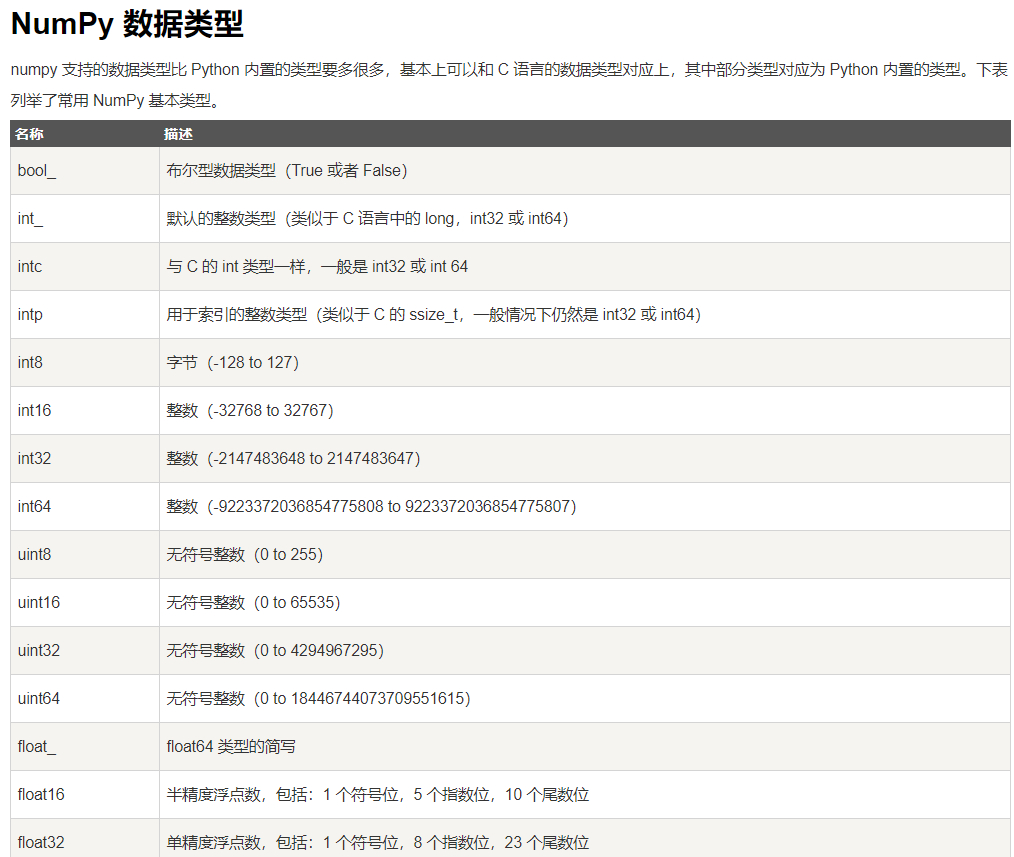### Python 的整数与 Numpy 的数据溢出

• 2019 年 10 月 3 日
• 筆記• Python 3 中整数的上限是多少？Python 2 呢？
• Numpy 中整数的上限是多少？出现整数溢出该怎么办？

• 一种是短整数，也即常说的整数，用 int 表示，有个内置函数 int()。其大小有限，可通过`sys.maxint()` 查看（取决于平台是 32 位还是 64 位）
• 一种是长整数，即大小无限的整数，用 long 表示，有个内置函数 long()。写法上是在数字后面加大写字母 L 或小写的 l，如 1000L

PEP-237（Unifying Long Integers and Integers）中对这个转变作了说明。它解释这样做的 目的：

Python 在语言运用层屏蔽了很多琐碎的活，比如内存分配，所以，我们在使用字符串、列表或字典等对象时，根本不用操心。整数类型的转变，也是出于这样的便利目的。（坏处是牺牲了一些效率，在此就不谈了）

``import numpy as np    a = np.arange(2)  type(a)    # 结果：numpy.int32``

Numpy 支持的数据类型要比 Python 的多，相互间的区分界限很多样：``import numpy as np    q =   w =     # 一个溢出的例子：  a = np.array(q)  b = np.array(w)  print(a*b)  # 产生溢出，结果是个奇怪的数值    # 一个解决的例子：  c = np.array(q, dtype='int64')  d = np.array(w, dtype='int64')  print(c*d) # 没有溢出：``

• Python 3 极大地简化了整数的表示，效果可表述为：整数就只有一种整数（int），没有其它类型的整数（long、int8、int64 之类的）
• Numpy 中的整数类型对应于 C 语言的数据类型，每种“整数”有自己的区间，要解决数据溢出问题，需要指定更大的数据类型（dtype）